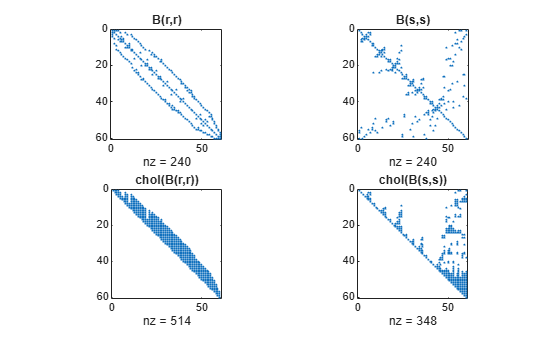symamd

Symmetric approximate minimum degree permutation

Syntax

p = symamd(S)
p = symamd(S,knobs)
[p,stats] = symamd(...)

Description

p = symamd(S) for a symmetric positive definite matrix S, returns the permutation vector p such that S(p,p) tends to have a sparser Cholesky factor than S. To find the ordering for S, symamd constructs a matrix M such that spones(M'*M) = spones (S), and then computes p = colamd(M). The symamd function may also work well for symmetric indefinite matrices.

S must be square; only the strictly lower triangular part is referenced.

p = symamd(S,knobs) where knobs is a scalar. If S is n-by-n, rows and columns with more than knobs*n entries are removed prior to ordering, and ordered last in the output permutation p. If the knobs parameter is not present, then knobs = spparms('wh_frac').

[p,stats] = symamd(...) produces the optional vector stats that provides data about the ordering and the validity of the matrix S.

 stats(1) Number of dense or empty rows ignored by symamd stats(2) Number of dense or empty columns ignored by symamd stats(3) Number of garbage collections performed on the internal data structure used by symamd (roughly of size 8.4*nnz(tril(S,-1)) + 9n integers) stats(4) 0 if the matrix is valid, or 1 if invalid stats(5) Rightmost column index that is unsorted or contains duplicate entries, or 0 if no such column exists stats(6) Last seen duplicate or out-of-order row index in the column index given by stats(5), or 0 if no such row index exists stats(7) Number of duplicate and out-of-order row indices

Although, MATLAB® built-in functions generate valid sparse matrices, a user may construct an invalid sparse matrix using the MATLAB C or Fortran APIs and pass it to symamd. For this reason, symamd verifies that S is valid:

• If a row index appears two or more times in the same column, symamd ignores the duplicate entries, continues processing, and provides information about the duplicate entries in stats(4:7).

• If row indices in a column are out of order, symamd sorts each column of its internal copy of the matrix S (but does not repair the input matrix S), continues processing, and provides information about the out-of-order entries in stats(4:7).

• If S is invalid in any other way, symamd cannot continue. It prints an error message, and returns no output arguments (p or stats).

The ordering is followed by a symmetric elimination tree post-ordering.

Examples

collapse all

Here is a comparison of reverse Cuthill-McKee and minimum degree on the Bucky ball example mentioned in the symrcm reference page.

B = bucky+4*speye(60);
r = symrcm(B);
p = symamd(B);
R = B(r,r);
S = B(p,p);
subplot(2,2,1), spy(R,4), title('B(r,r)')
subplot(2,2,2), spy(S,4), title('B(s,s)')
subplot(2,2,3), spy(chol(R),4), title('chol(B(r,r))')
subplot(2,2,4), spy(chol(S),4), title('chol(B(s,s))')Even though this is a very small problem, the behavior of both orderings is typical. RCM produces a matrix with a narrow bandwidth which fills in almost completely during the Cholesky factorization. Minimum degree produces a structure with large blocks of contiguous zeros which do not fill in during the factorization. Consequently, the minimum degree ordering requires less time and storage for the factorization.

References

The authors of the code for symamd are Stefan I. Larimore and Timothy A. Davis (davis@cise.ufl.edu), University of Florida. The algorithm was developed in collaboration with John Gilbert, Xerox PARC, and Esmond Ng, Oak Ridge National Laboratory. Sparse Matrix Algorithms Research at the University of Florida: https://www.cise.ufl.edu/research/sparse/